top of page
Search

# DAX: Data Modeling with RELATED & RELATEDTABLEIn data modeling, the relationship between tables plays a vital part. Just like in the physical world, if we build strong and meaningful relationships, it makes our lives easy.

Relationships in the data model add much flexibility, and two DAX functions thrive on this capability.

RELATED and RELATEDTABLE are two elementary but powerful functions. The critical difference between them is that RELATED works on the “many-side “ of the relationship, and RELATEDTABLE works on the “one-side” of the relationship.

Let us consider the following example:Model view:There is a many-to-one relationship between the dtScore and dtEmp tables:Sample Data file

## RELATED

Related DAX takes only one argument: ColumnName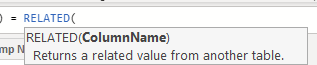### Objective 1: Add a Calculated Column with the name of the employee in the dtScore table

dtScore table is at the “many-side” of the relationship, so we use the RELATED function to add a calculated column

The moment we type RELATED, IntelliSense shows the list of all the fields related to the current table:Since we need the employee name, we select the column dtEmp[Name] from the suggestion.We had obtained the same results using LOOKUPVALUE:### Objective 2: Using RELATED with CALCULATE

We use the RELATED function when we have multiple dimension tables and one fact table (Star Schema). We can write some useful DAX measures using the CALCULATE and RELATED.

Let us consider the following data model: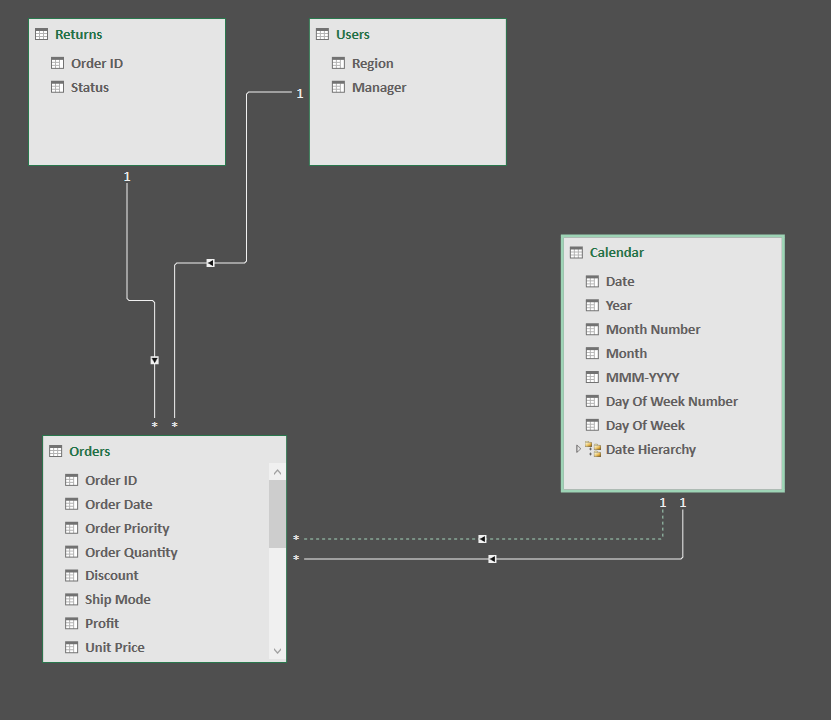Power Pivot Model

Power BI Desktop

Returns table contains a list of all the orders which have returned. We need to identify the sales amount of all the product which has returned. There is a many-to-one relationship between Orders and Returns table

We add the following DAX measures to the model:

``` Sales Amount:=
SUMX(
Orders,
Orders[Unit Price] * Orders[Order Quantity]
)

Returned Sales Amount =
//Filter the order table on order status = Returned
VAR _FilteredTable =
FILTER(
dtOrders,
RELATED(dtReturns[Status]) = "Returned"
)

VAR _RelatedSales =
SUMX(_FilteredTable,[Sales Amount])

RETURN
_RelatedSales

% Sales Refund:=
DIVIDE([Returned Sales Amt],[Sales Amount])

```

RELATED function needs a row context; hence we are using it with FILTER functions to create the row context.

Following is the output:Alternatively, we can write this code using the CALCULATE:

``` Returned Sales Amount (w Calculate) =
CALCULATE([Sales Amount],dtReturns[Status] = "Returned")
```

Using the DAX measures just created, we can analyze and identify where the challenges are.

## RELATEDTABLE

The main difference between RELATED and RELATEDTABLE is the direction of the relationship. RELATED functions on the “long” (many) side of the relationship. In contrast, RELATEDTABLE works on the “short”(one) side of the relationship.

RELATEDTABLE takes one argument: Table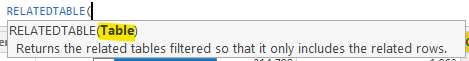In the current data model, there is a many-to-one relationship between the dtOrders and dtUser.### Objective: Add a calculated column with the sum of order quantity in the ftUsers table

``` Total Orders (cc) =
SUMX(
RELATEDTABLE(dtOrders),
dtOrders[Order Quantity]
)
```RELATEDTABLE function changes the context in which the data is filtered and evaluates the function in the new specified context, just like using CALCULATE or CALCULATETABLE. The RELATEDTABLE function is equivalent to CALCULATETABLE minus the logical/filter expressions.

In the above example, we have used RELATEDTABLE function in a calculated column; hence it is working in the row context. If we recall from the article FILTER & ROW Context, the calculated column automatically applies the row context during evaluation.

Following example may help in understanding the difference:

I have added another calculated column without using RELATEDTABLE:

``` Total Orders (SUMX,CC) =
SUMX(
dtOrders,
dtOrders[Order Quantity]
)
```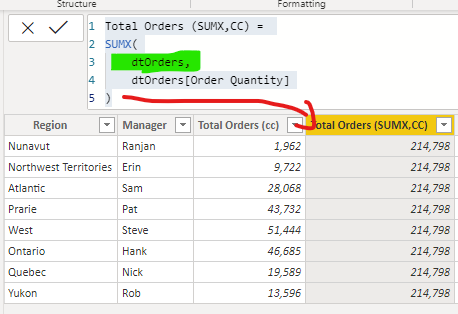And when I wrap the above formula in CALCULATE, it enables the context transition:

``` Total Orders (Calcualte,CC) =
CALCULATE(
SUMX(
dtOrders,
dtOrders[Order Quantity]
)
)
```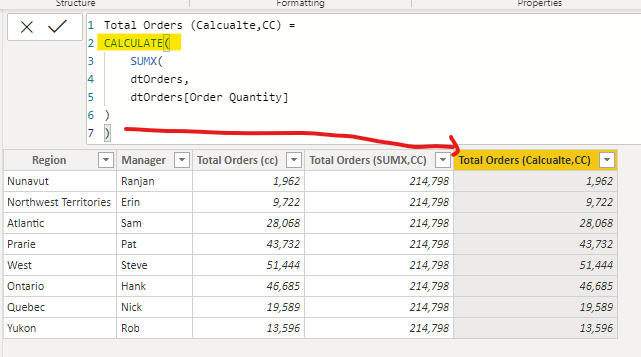Notice we get the exact same result with RELATEDTABLE.

### Using RELATEDTABLE in DAX measure

We can use the following measure to calculate the total sales amount of the orders which has returned:

``` Returned Sales (RelatedTable) =
SUMX(
RELATEDTABLE(dtReturns),
[Sales Amount]
)
```

Using RELATEDTABLE, we are filtering the dtOrders table where it has the matching Order ID in the dtReturn table, and then running the DAX measure for [Sales Amount].If we have established the right relationship, we can unleash the power of DAX.

@imVivRan

bottom of page